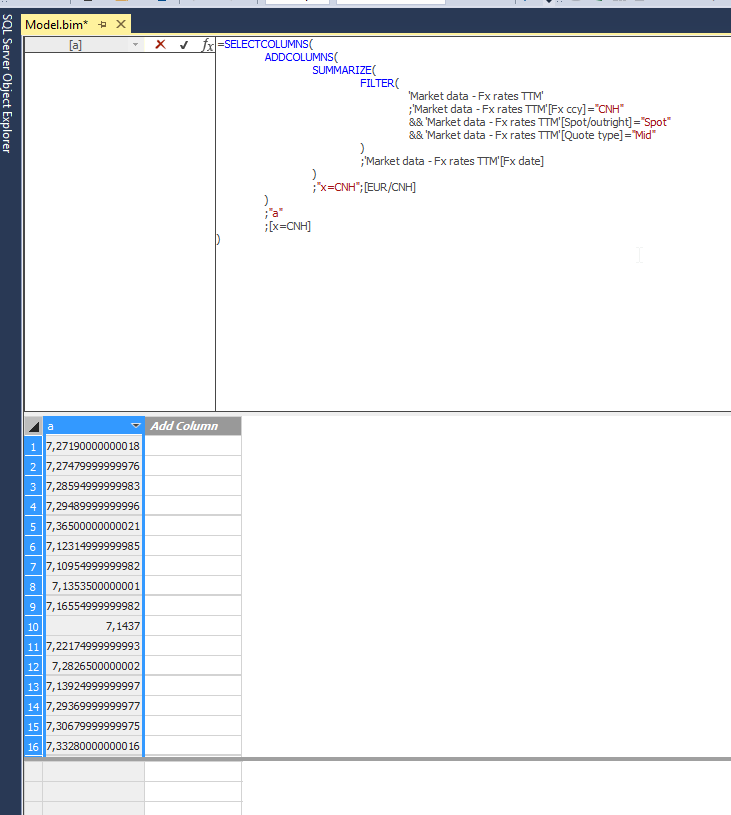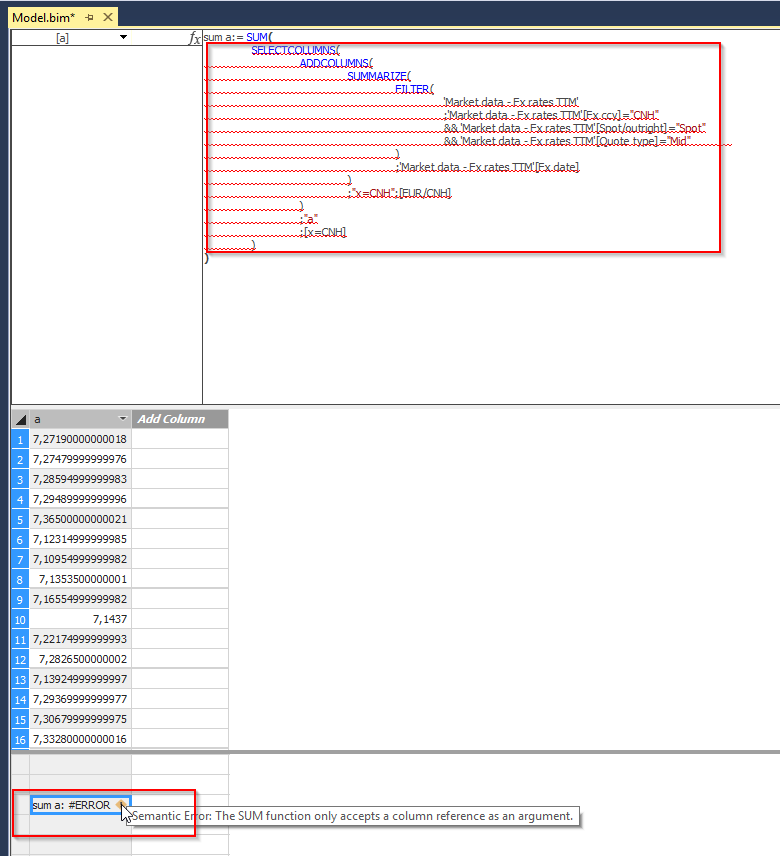cancel
Showing results for
Did you mean:Helper II

## SUM only accepts column ref

Hi there

I have DAX code for a one-column ("a") calculated table. See code and screenshot below.

```SELECTCOLUMNS(
SUMMARIZE(
FILTER(
'Market data - Fx rates TTM'
;'Market data - Fx rates TTM'[Fx ccy]="CNH"
&& 'Market data - Fx rates TTM'[Spot/outright]="Spot"
&& 'Market data - Fx rates TTM'[Quote type]="Mid"
)
;'Market data - Fx rates TTM'[Fx date]
)
;"x=CNH";[EUR/CNH]
)
;"a"
;[x=CNH]
)```What I want to do is SUM the values of this column, i.e. Measure = sum(calculated_table[a]).

BUT, I want to do this all _in memory_, without the need of an intermediate calculated table... therefore I went ahead and put this code into a SUM function directly

```sum a:= SUM(
SELECTCOLUMNS(
SUMMARIZE(
FILTER(
'Market data - Fx rates TTM'
;'Market data - Fx rates TTM'[Fx ccy]="CNH"
&& 'Market data - Fx rates TTM'[Spot/outright]="Spot"
&& 'Market data - Fx rates TTM'[Quote type]="Mid"
)
;'Market data - Fx rates TTM'[Fx date]
)
;"x=CNH";[EUR/CNH]
)
;"a"
;[x=CNH]
)
)```However, this throws an error, stating that sum only takes a column reference...

Kind regards

1 ACCEPTED SOLUTIONCommunity Champion

2 REPLIES 2Community ChampionHelper II

@Sean Thank you! Didn't think of that!

Cheers